{{announcement.body}}
{{announcement.title}}

# Time Series Data Analysis With R, Part 1

DZone 's Guide to

# Time Series Data Analysis With R, Part 1

### In this post, we use R and few of its packages to develop time series data analyses and visualizations.

· Big Data Zone ·
Free Resource

Comment (0)

Save
{{ articles.views | formatCount}} Views

R provides a number of different packages and built-in functions that make it possible to effortlessly work with time series data. Time series data is essentially a retrospective, looking back at a stream of events to derive insights into which past event had a lasting impact compared to another, or due to a chain of such events.

We will use the J&J dataset available with the astsa package in R.  Let's explore how it looks:

``````> jj
Qtr1      Qtr2      Qtr3      Qtr4
1960  0.710000  0.630000  0.850000  0.440000
1961  0.610000  0.690000  0.920000  0.550000
1962  0.720000  0.770000  0.920000  0.600000
1963  0.830000  0.800000  1.000000  0.770000
1964  0.920000  1.000000  1.240000  1.000000
1965  1.160000  1.300000  1.450000  1.250000
1966  1.260000  1.380000  1.860000  1.560000``````

So this is J&J quarterly EPS data over 84 quarters begining 1960.

Let's visualize this data to see any trends and seasonality?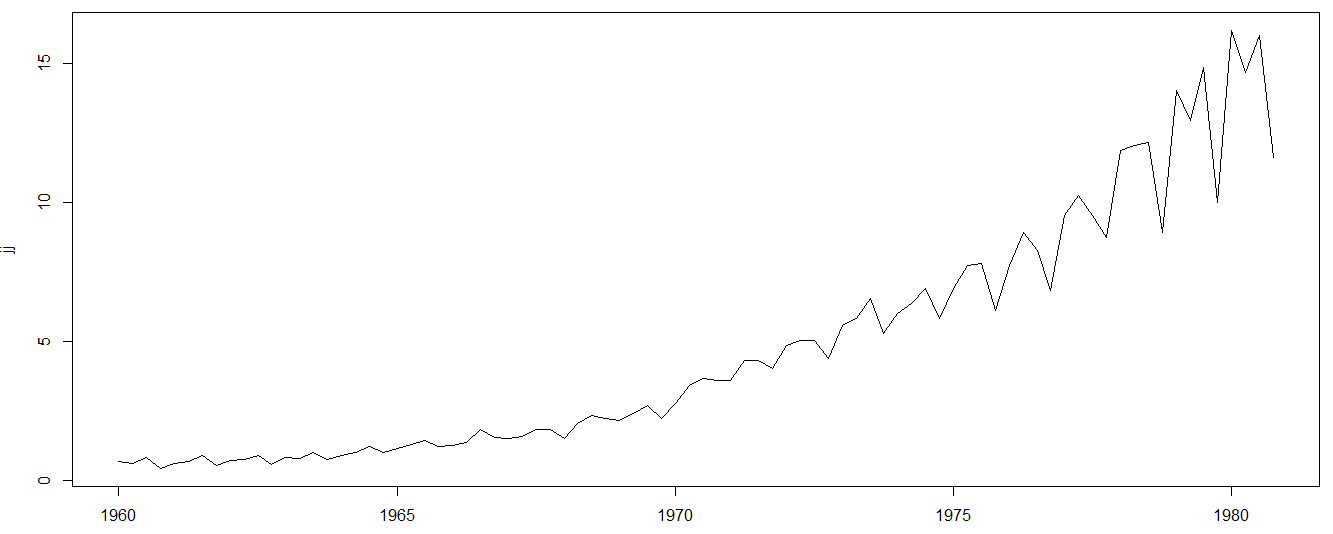Quite obviously, a visual scan tends to identify the fluctuations, repetitions, and overall trends.

An analytical way would be to decompose these into separate components to see the impact of each.

Before doing that, let's try to answer the basic question: What is constant among the chaos and what transformations are there to discover?

This can be a trial and error approach, where validation tests such as Dicky-Fuller are used to come to a conclusion.

But, instinctively, you tend to compare the difference, and perhaps a `diff(log(X))` is good enough to try.

``plot(diff(log(jj)))``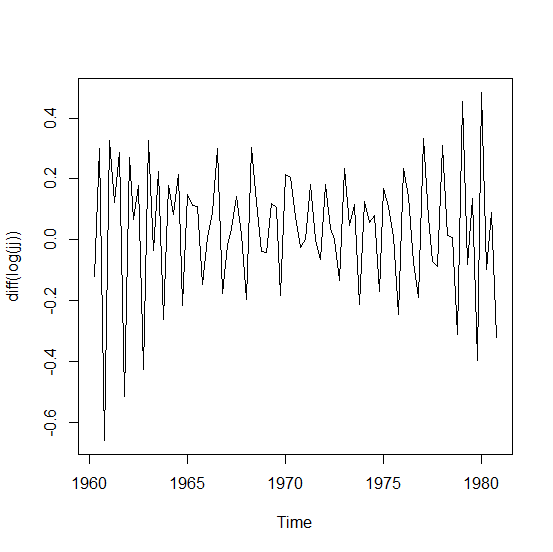So this is perhaps the change that was keeping itself stationary in the overall set of observations.

This hypothesis must, of course, be validated by statistical tests.

Let's run a Dicky-Fuller test to do so.

``````##Dicky-Fuller Test
Augmented Dickey-Fuller Test

data:  diff(log(jj))
Dickey-Fuller = -4.5649, Lag order = 4, p-value = 0.01
alternative hypothesis: stationary

Warning message:
In adf.test(diff(log(jj)), alternative = "stationary") :
p-value smaller than printed p-value``````

So, this validates our initial hypothesis about any stationary behavior in the data, as we must reject the null hypothesis and embrace the alterative.

We can also visualize past values (lagging) plotted relative to the present values.

``lag1.plot(jj, 6) ``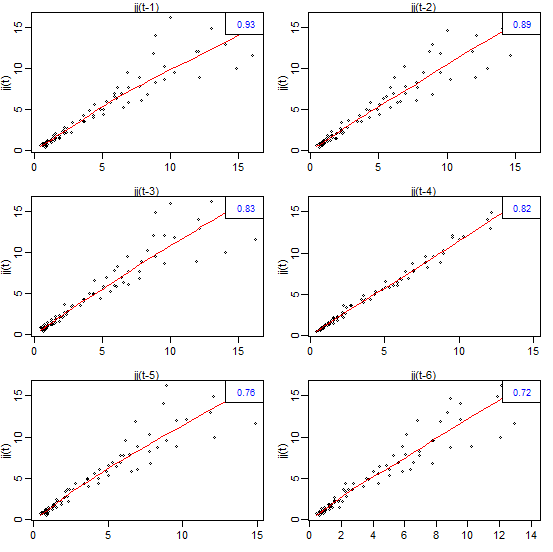You can see in above example that the pastsix lagging values seem to be linearly proportional to the present values.

Now, let us again look at the impact of the difflog transformation through the same visualization.You can see that the plotted values tend to be more flat (signalling a stationary nature) than linear, except for one or two specific lags.

Thinking about linear approximation (the red line), let's explore if it is possible for us to assign specific weights to lagging values to model the approximation. This is about the model trying to rise above the fluctuations and begining to see larger trends (upward or downward).

``lines(filter(jj, sides = 2,method='c', c(0.25,0.50,0.50,0.50,0.25)),col='green')``

The model is looking on either side (`sides=2`) of the present value (at any instant). So its looking forward and backward in time (as far as dataset values are concerned) and assigns weights according to the specified numeric vectors.

Here is how the model sees the time series in its own simplification as it rides on the trends to see the overall trend. Perhaps a bit steeper than reality....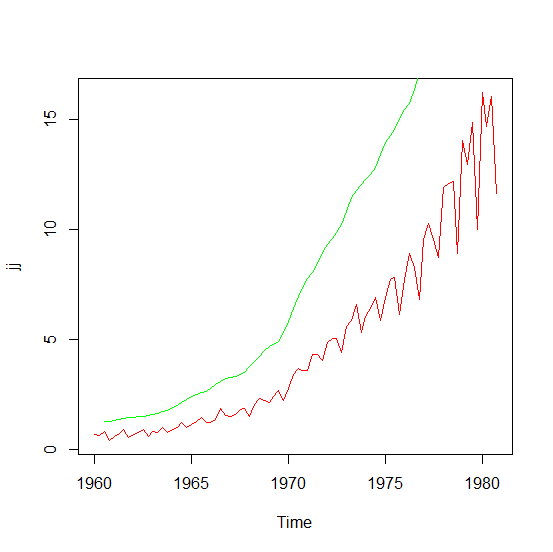Let's make the model does not rely on the weights provided to it, but makes its own calculations using the moving averages.

``lines(ma(jj, order=5), col="blue", lty="dashed")``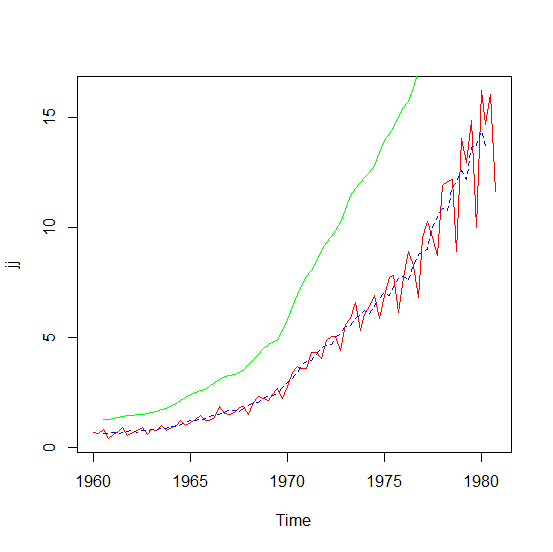Now, the model is swimming alongside the time series, rather than solely relying on weights.

We can also try lowess, which is a much more complex algorithm implemented in the Stats package for R. For more details, see library/stats/html/lowess.html in your installation.

``lines(lowess(jj), col="magenta")``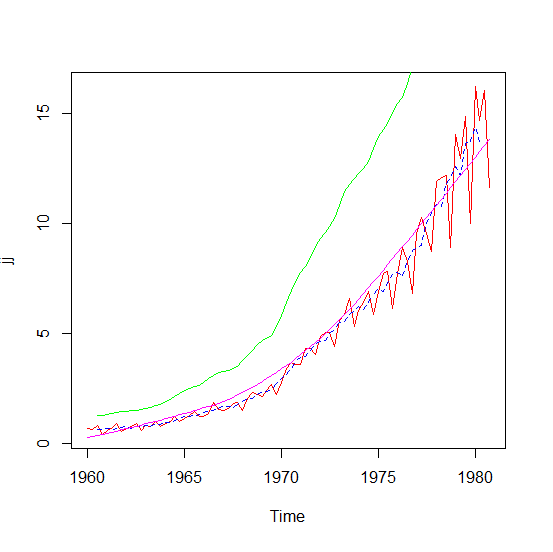This time the trend line (the magenta color) rides over the tips, rather than dipping and rising as per the moving average. This is, again, symmetrical around a given value, depending on the order value specified, where the symmetric interval for looking forward and backward is `(order-1)/2`.

Coming back to our analytical way of decomposing the trend, let us try to do so using the `decompose` method in R. The objective is to see through the trends, seasonality, and random errors.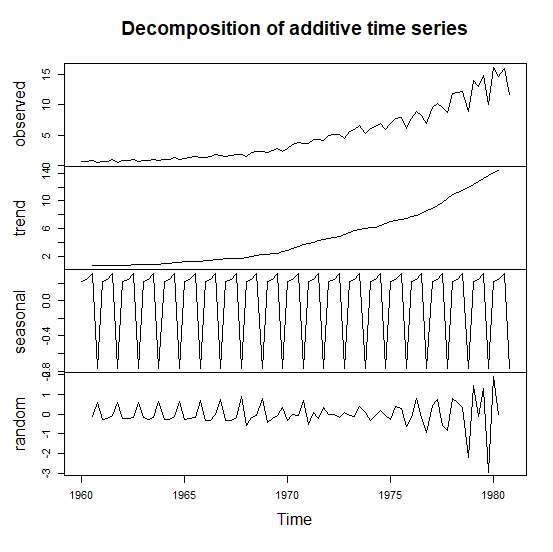Similarly, we can decompose the "stationary" transformation.

``plot(decompose(diff(log(jj)),type="additive"))``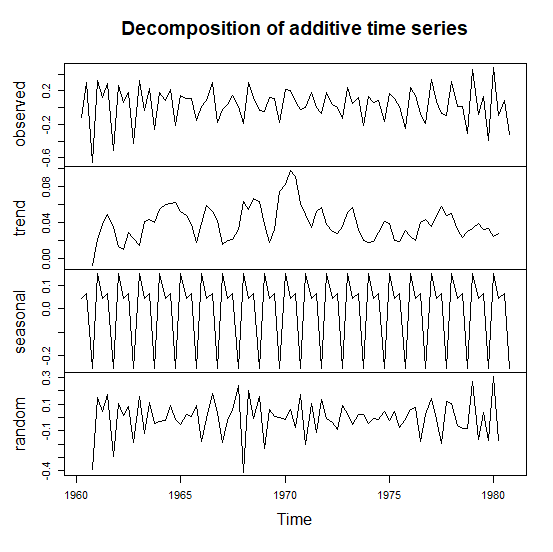We will explore more about trends and seasonality and how we can form ARIMA (Autoregressive, Integrated, Moving Average) models in the next article.

Topics:
big data, r tutorials, r tutorials for beginners, time series data

Comment (0)

Save
{{ articles.views | formatCount}} Views

Opinions expressed by DZone contributors are their own.